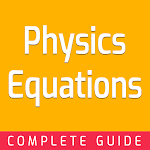ID: com.dotpro.physicsequations

## The description of Physics Equations

Physics equations app is for beginners. This Physics equations app will provide you definition and example. If you are looking for basic Physics equations so you are in a right place. This application will give you example and explanation.

This basic Physics equations app is essential for students. This Physics equations app will help you to learn free and offline. It will make your learning easier than before. So now you can carry your physics formula collection with you anywhere by this app and can learn anytime.

Learn Physics equations app feature:
- Definition & classification
- Example & explanation
- Picture example
- Detail information & explanation
- Chapter based physics formula collection
- Basic Physics equations offline
- User friendly
- Works without internet
- Optimized for mobile & tablets

Learn Physics equations app will provide you most important & informative lessons. This basic Physics equations will give you step by step guideline. It will make your learning easier. This Physics formula collection will help you to learn quickly through an app. Hope you will like this Physics equations app and learn from it. So keep install and learn.